地球物理学报2016, Vol. 59Issue (8): 3098-3109PDF

1. 中南大学地球科学与信息物理学院, 长沙 410083;
2. 有色资源与地质灾害探查湖南省重点实验室, 长沙 410083

The GPR simulation of bi-phase random concrete medium using finite element of B-spline wavelet on the interval
FENG De-Shan1,2, WANG Xun1,2
1. School of Geosciences and Info-Physics, Central South University, Changsha 410083, China;
2. Key Laboratory of Non-ferrous Resources and Geological Detection, Ministry of Hunan Province, Changsha 410083, China
Abstract: Based on the separable wavelet theory, the scaling functions of one-dimensional B-spline wavelet on the interval (BSWI) is employed to construct the two-dimensional B-spline wavelet bases on the interval. In order to solve the GPR wave equation, these constructed wavelet bases are used as the interpolation functions, and a transformation matrix is introduced to convert between the wavelet coefficient space and the physical space (radar electromagnetic field). In this study, the discrete format of GPR wave equation for two-dimensional interval B-spline wavelet finite element is derived using Galerkin algorithm. And the integral values and the connection coefficients of the second order one-scale and second order two-scale BSWI functions are calculated. The detailed process of the algorithm is given. Then two typical examples are forward modeled using the BSWI method by Matlab program. The result shows that the BSWI with fewer units has the same precision as FEM. Increasing the scale of BSWI algorithm, the precision of the results is also improved, but it is time-consuming. Finally, the BSWI algorithm is applied to model bi-phase random concrete medium, and it proves that the random medium model theory can describe the practical distribution of concrete medium flexibly and effectively. Furthermore, this study found that the forward modeling profile is more accordant with practical profile, which demonstrates that medium model theory can simulate the transmission process of the radar wave more accurately. It also provides a theoretical basis for improving the detection results and interpretation accuracy of GPR..
Key words: Ground Penetrating Radar      B-spline wavelet on the interval      Wavelet finite element method      Forward modeling      Bi-phase random medium
1 引言

2 区间B样条小波特性及二维张量积小波构造 2.1 区间B样条小波性质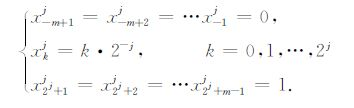(1)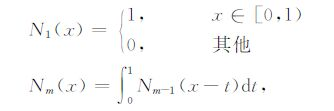(2)

m阶B样条尺度函数表示为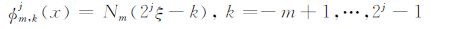(3)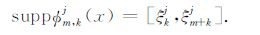(4)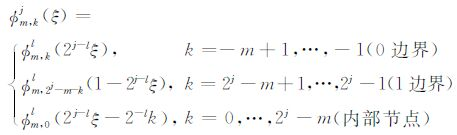(5)

BSWI21尺度函数有3个，其中0边界φ2－11，(ξ)，1边界尺度函数φ2，11(ξ)，内部尺度函数φ2，01(ξ)，即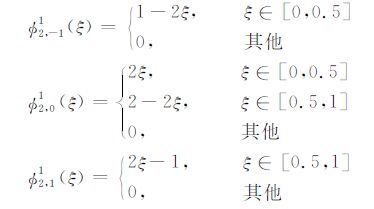(6)

BSWI22尺度函数有5个，其中0边界φ2，－12(ξ)，1边界尺度函数φ2，32(ξ)，内部尺度函数φ2，02(ξ)，φ2，12(ξ)，φ2，22(ξ)，即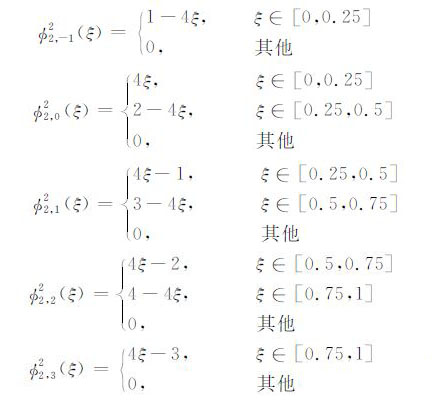(7)

2.2 二维张量积小波构造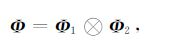(8)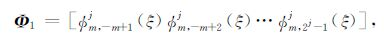(9)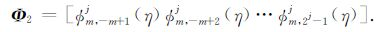(10)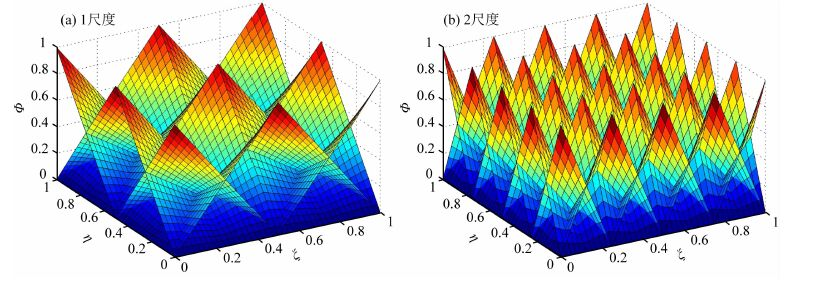图 1 二维张量积BSWI2尺度函数图 Fig. 1 The map of two-dimensional tensor product scaling function of BSWI2
3 GPR波动方程的区间B样条小波有限元求解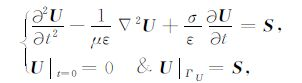(11)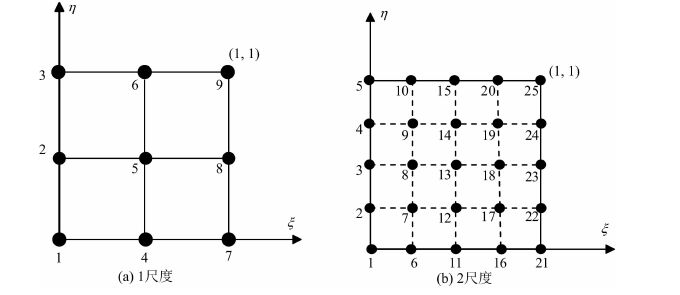图 2 二维BSWI2小波单元 Fig. 2 Two-dimensional BSWI2 wavelet element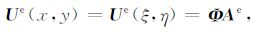(12)

Ue为单元内的电场值或磁场值，x，y为总体坐标系，ζ，η为局部坐标系.局部坐标与总体坐标的转换关系为：x=a·ζy=b·ηab分别为1个单元的长与宽.其中Ae表示单元上待求的小波插值系数列向量，表示为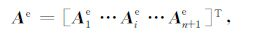(13)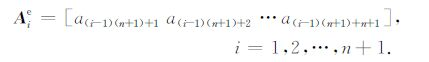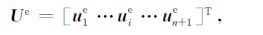(14)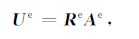(15)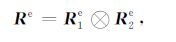(16)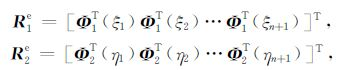(17)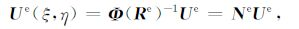(18)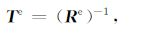(19)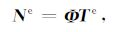(20)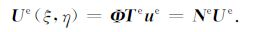(21)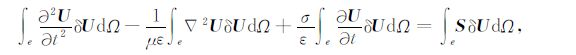(22)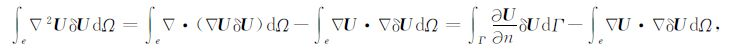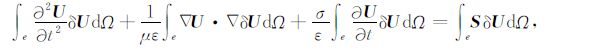(23)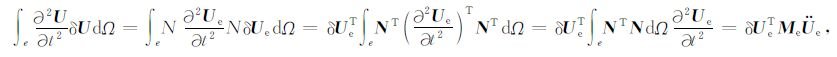(24)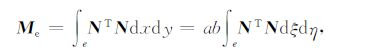(25)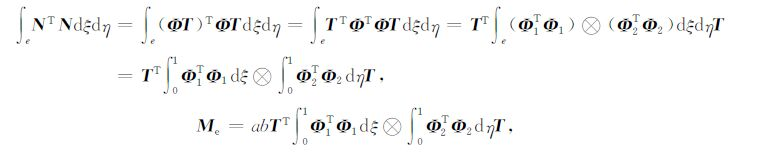(26)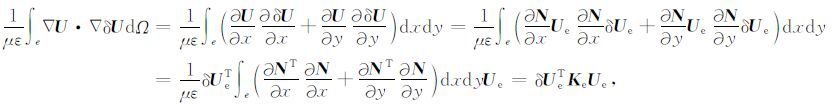(27)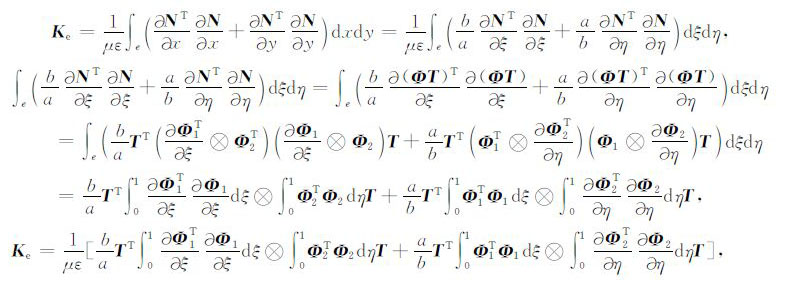(28)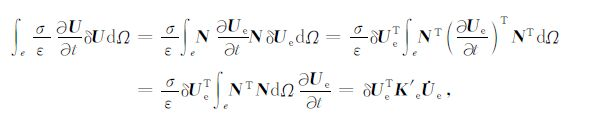(29)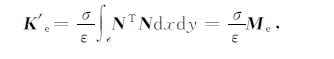(30)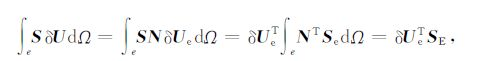(31)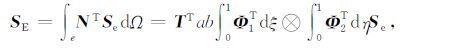(32)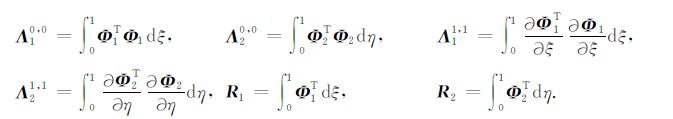(33)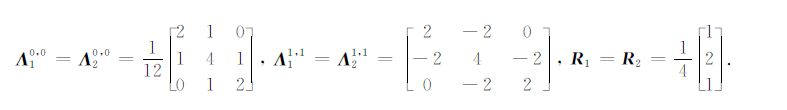2尺度：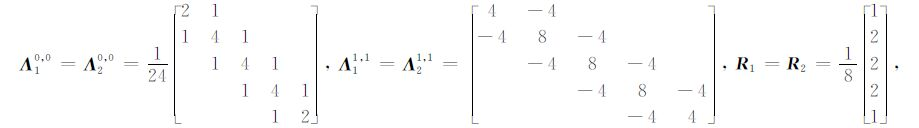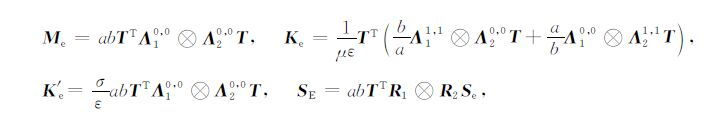(34)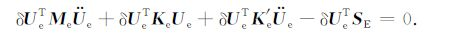(35)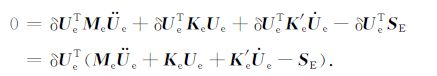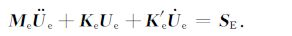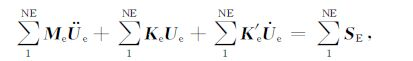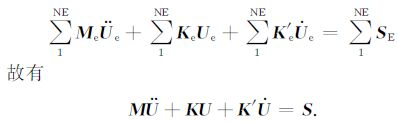(36)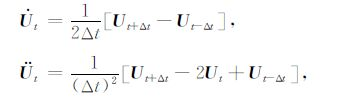(37)

(36)式可化为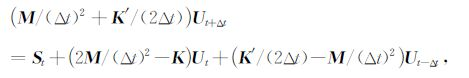(38)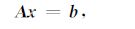(39)

4 BSWI算法实例分析 4.1 模型一 BSWI与FEM对比试验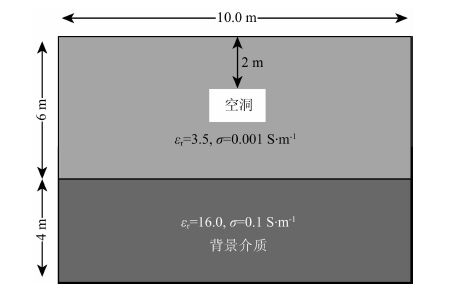图 3 雷达模型一示意图 Fig. 3 The sketch map of GPR model 1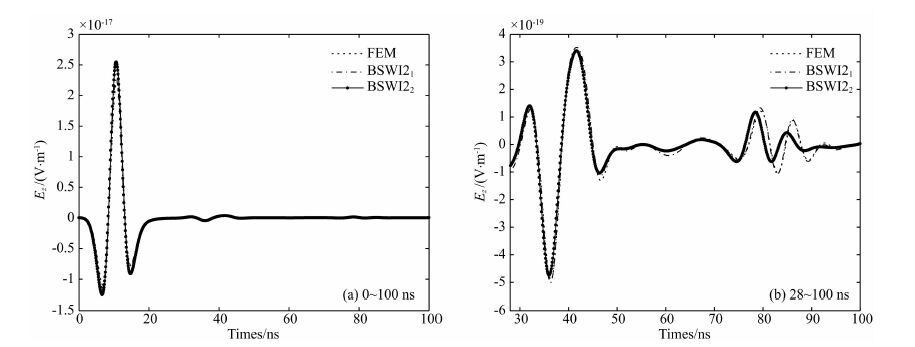图 4 有限元、BSWI21 及BSWI22波形数据对比 Fig. 4 Contrasting between FEM BSWI21 and BSWI22 wavelet FEM of GPR waveform

4.2 模型二 BSWI21与BSWI22尺度提升前后对比试验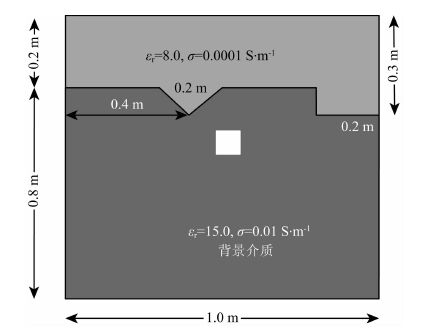图 5 雷达模型二示意图 Fig. 5 The sketch map of GPR model 2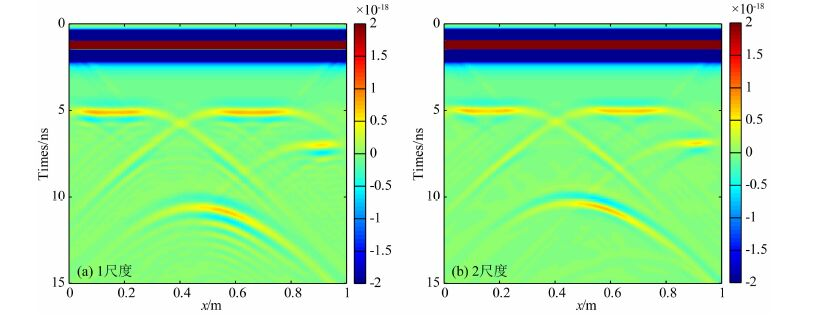图 6 模型二雷达正演剖面图 Fig. 6 The section of GPR simulation

5 双相随机介质模型数值算例 5.1 双相随机介质建模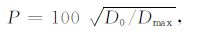(40)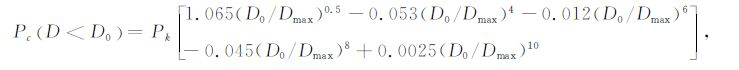(41)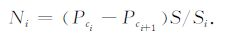(42)

(1) 根据级配曲线计算各粒径级颗粒数；

(2) 依据粒级参数，依次随机生成椭圆长轴，短轴，形心坐标以及倾角参数；

(3) 判断是否与已有椭圆骨料干涉，若发生干涉返回步骤(2)；

(4) 输出该骨料参数；

(5) 判断该粒级是否完成，若未完成返回步骤(2)；

(6) 判断是否存在下一粒级，若存在，改变粒级参数并返回步骤(2)；

(7) 椭圆骨料生成完毕.

5.2 混凝土模型BSWI模拟算例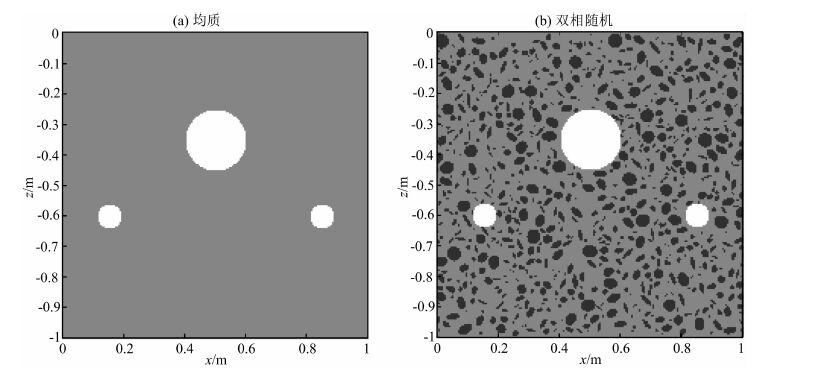图 7 雷达模型图三 Fig. 7 The sketch map of GPR model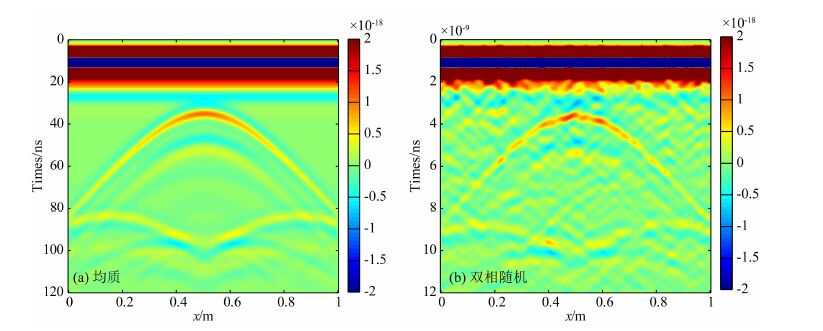图 8 模型三雷达正演剖面图 Fig. 8 The section of model 3 GPR simulation
6 结论

(1) 编制了二维区间B样条小波有限元GPR正演程序，通过对比相同的剖分方式及节点数目条件下GPR单道波形图，BSWI算法能以较少的计算时间达到FEM相似的精度，证明BSWI算法的正确性.而将BSWI的尺度提升后，模拟得到的雷达波形更简洁、能更清晰地体现异常体信息，对解的精度有较为明显的提高，但计算时间大大增加.说明BSWI是区别于传统网格法和多项式法的另一种提高精度的细化方法，能在不改变网格剖分的前提下提高分辨率，为GPR波动方程求解提供新的思路.

(2) 将混凝土视为随机介质，构建了双相离散随机混凝土模型，使其在物质组成材料参数、骨料形态及分布上与真实的混凝土更具一致性.采用BSWI方法模拟了双相离散随机模型，与均质介质GPR正演剖面图相比，随机介质中GPR波形散射严重，波形扭曲，异常体绕射波弧形欠光滑、波形振幅较弱、剖面中出现了较多的干扰杂波.但随机介质模型的正演剖面能更真实地模拟雷达波的传播过程，与实测剖面特征更相符，更有利于指导雷达剖面的数据解译.

 Amaratunga K, Williams J R. 1993. Wavelet based Green's function approach to 2D PDES. Engineering Computations , 10(4): 349–367. Amaratunga K, Williams J R, Qian S, et al. 1994. Wavelet-Galerkin solutions for one-dimensional partial differential equations. International Journal for Numerical Methods in Engineering , 37(16): 2703–2716. Chen X F, Yang S J, Ma J X, et al. 2004. The construction of wavelet finite element and its application. Finite Elements in Analysis and Design , 40(5-6): 541–554. Chen X F, He Z J, Xiang J W, et al. 2006. A dynamic multiscale lifting computation method using Daubechies wavelet. Journal of Computational and Applied Mathematics , 188(2): 228–245. Chen X F, Xiang J W, Li B, et al. 2010. A study of multiscale wavelet-based elements for adaptive finite element analysis. Advances in Engineering Software , 41(2): 196–205. Chui C K, Quak E. 1992. Wavelets on a bounded interval.//Numerical Methods in Approximation Theory, Vol.9. Birkhäuser Basel, 53-75. Dai Q W, Wang H H. 2013. Element free method forward modeling of GPR based on random medium model. The Chinese Journal of Nonferrous Metals (in Chinese) , 23(9): 2436–2443. Daubechies I. 1998. Orthonormal bases of compactly supported wavelets. Communications on Pure and Applied Mathematics , 41(7): 909–996. Di Q Y, Wang M Y. 1999. 2D finite element modeling for radar wave. Chinese J. Geophys. (in Chinese) , 42(6): 818–825. Di Q Y, Wang M Y. 2004. Migration of ground-penetrating radar data with a finite-element method that considers attenuation and dispersion. Geophysics , 69(2): 472–477. Feng D S, Chen C S, Dai Q W. 2010. GPR numerical simulation of full wave field based on UPML boundary condition of ADI-FDTD. Chinese J. Geophys. , 53(10): 2484–2496. doi: 10.3969/j.issn.0001-5733.2010.10.022. Feng D S, Chen C S, Wang H H. 2012. Finite element method GPR forward simulation based on mixed boundary condition. Chinese J Geophys. , 55(11): 3774–3785. doi: 10.6038/j.issn.0001-5733.2012.11.024. Gao Z G, Liu G T. 2003. Two-dimensional random aggregate structure for concrete. Journal of Tsinghua University(Science and Technology) (in Chinese) , 43(5): 710–714. Goswami J C, Chan A K, Chui C K. 1995. On solving first-kind integral equations using wavelets on a bounded interval. IEEE Transactions on Antennas and Propagation , 43(6): 614–622. Guo S L. 2013. Research on GPR wave theory in random medium for detecting highway[Ph. D. thesis] (in Chinese). Wuhan:China University of Geosciences. He Z J, Chen X F, Li B, et al. Theory of the Wavelet Based Finite Element Methods and the Application in Engineering . (in Chinese) Beijing: Science Press, 2006 . Jin J M, Xu Y X, Xue P X. 2006. Minimum support spline wavelet finite element. Mathematica Numerica Sinica (in Chinese) , 28(1): 89–112. Li J, Zeng Z F, Wu F S, et al. 2010. Study of three dimension high-order FDTD simulation for GPR. Chinese J. Geophys. , 53(4): 974–981. doi: 10.3969/j.issn.0001-5733.2010.04.022. Li J. 2014. Ground penetrating radar detection and parameter inversion in stochastic effective medium[Ph. D. thesis] (in Chinese). Changchun:Jilin University. Liang X B, Jian B D, Ni G Z. 1988. The B-spline finite element method for electromagnetic field analysis. Journal of Zhejiang University (in Chinese) , 22(2): 100–110. Liu S X, Zeng Z F. 2007. Numerical simulation for Ground Penetrating Radar wave propagation in the dispersive medium. Chinese J. Geophys. (in Chinese) , 50(1): 320–326. Mishra V, Sabina. 2011. Wavelet Galerkin solutions of ordinary differential equations. Int. J. Math. Anal. , 5(9-12): 407–424. Patton R D, Marks P C. 1996. One-dimensional finite elements based on the Daubechies family of wavelets. AIAA Journal , 34(8): 1696–1698. Quak E, Weyrich N. 1994. Decomposition and reconstruction algorithms for spline wavelets on a bounded interval. Applied and Computational Harmonic Analysis , 1(3): 217–231. Sabina, Mishra V. 2012. Wavelet-Galerkin solutions of one and two dimensional partial differential equations. Journal of Emerging Trends in Computing and Information Sciences , 3(10): 1373–1378. Sarkar T K, Adve R S, García-Castillo L E, et al. 1994. Utilization of wavelet concepts in finite elements for an efficient solution of Maxwell's equations. Radio Science , 29(4): 965–977. Shih C T. 1979. On spline finite element method. Mathematica Numerica Sinica (in Chinese) , 1(1): 50–72. Sun H X, Xu J Y, Kang T, et al. 2014. The three-dimension spline wavelet finite element construction and application on antiknock of underground box structure. Journal of Xi'an University of Architecture & Technology (Natural Science Edition) , 46(6): 816–821. Walraven J C, Reinhardt H W. 1981. Theory and experiments on the mechanical behaviour of cracks in plain and reinforced concrete subjected to shear loading. Heron , 26(1A): 26–35. Xiang J W, Chen X F, He Z J, et al. 2007. The construction of 1D wavelet finite elements for structural analysis. Computational Mechanics , 40(2): 325–339. Xiang J W, Chen X F, He Z J, et al. 2008a. A new wavelet-based thin plate element using B-spline wavelet on the interval. Computational Mechanics , 41(2): 243–255. Xiang J W, Chen X F, Yang L F, et al. 2008b. A class of wavelet-based flat shell elements using B-spline wavelet on the interval and its applications. CMES-Computer Modeling in Engineering and Sciences , 23(1): 1–12. Xu S Z. The Finite Element Method in Geophysics . (in Chinese) Beijing: Science Press, 1994 . Yee K S. 1966. Numerical solution of initial boundary value problems involving Maxwell's equations in isotropic media. IEEE Transactions on Antennas and Propagation , 14(3): 302–307. Yu T. 2013. Numerical simulation of ultrasonic wave propagation in concrete[Master thesis] (in Chinese). Changsha:Central South University. Zhang J, Jin N G, Jin X Y, et al. 2004. Numerical simulation method for polygonal aggregate distribution in concrete. Journal of Zhejiang University (Engineering Science) (in Chinese) , 38(5): 581–585. Zhang X M, Liu K A, Liu J Q. 2005. A wavelet finite element method for the 2-D wave equation in fluid-saturated porous media. Chinese J Geophys. (in Chinese) , 48(5): 1156–1166. Zhong G Q, Li L J, Liu F, et al. 2008. Numerical simulation of two-dimensional random aggregate in concrete. Concrete (in Chinese) (9): 70–73. 戴前伟, 王洪华. 2013. 基于随机介质模型的GPR无单元法正演模拟. 中国有色金属学报 , 23(9): 2436–2443. 底青云, 王妙月. 1999. 雷达波有限元仿真模拟. 地球物理学报 , 42(6): 818–825. 冯德山, 陈承申, 戴前伟. 2010. 基于UPML边界条件的交替方向隐式有限差分法GPR全波场数值模拟. 地球物理学报 , 53(10): 2484–2496. 冯德山, 陈承申, 王洪华. 2012. 基于混合边界条件的有限单元法GPR正演模拟. 地球物理学报 , 55(11): 3774–3785. 高政国, 刘光廷. 2003. 二维混凝土随机骨料模型研究. 清华大学学报(自然科学版) , 43(5): 710–714. 郭士礼. 2013. 基于随机介质的高速公路探地雷达检测理论研究[博士论文]. 武汉:中国地质大学. 何正嘉, 陈雪峰, 李兵, 等. 小波有限元理论及其工程应用. 北京: 科学出版社, 2006 . 金坚明, 徐应祥, 薛鹏翔. 2006. 最小支集样条小波有限元. 计算数学 , 28(1): 89–112. 李静, 曾昭发, 吴丰收, 等. 2010. 探地雷达三维高阶时域有限差分法模拟研究. 地球物理学报 , 53(4): 974–981. 李静. 2014. 随机等效介质探地雷达探测技术和参数反演[博士论文]. 长春:吉林大学. 梁旭彪, 简柏敦, 倪光正. 1988. 电磁场数值分析中的B样条有限元法. 浙江大学学报 , 22(2): 100–110. 刘四新, 曾昭发. 2007. 频散介质中地质雷达波传播的数值模拟. 地球物理学报 , 50(1): 320–326. 石钟慈. 1979. 样条有限元. 计算数学 , 1(1): 50–72. 孙惠香, 许金余, 康婷, 等. 2014. 三维样条小波单元构造及其在地下箱型结构抗爆数值模拟中的应用. 西安建筑科技大学学报(自然科学版) , 46(6): 816–821. 徐世浙. 地球物理中的有限单元法. 北京: 科学出版社, 1994 . 余涛. 2013. 超声波在混凝土中传播的数值模拟[硕士论文]. 长沙:中南大学. 张剑, 金南国, 金贤玉, 等. 2004. 混凝土多边形骨料分布的数值模拟方法. 浙江大学学报(工学版) , 38(5): 581–585. 张新明, 刘克安, 刘家琦. 2005. 流体饱和多孔隙介质二维弹性波方程正演模拟的小波有限元法. 地球物理学报 , 48(5): 1156–1166. 钟根全, 李丽娟, 刘锋, 等. 2008. 混凝土二维随机骨料的数值模拟. 混凝土 (9): 70–73.